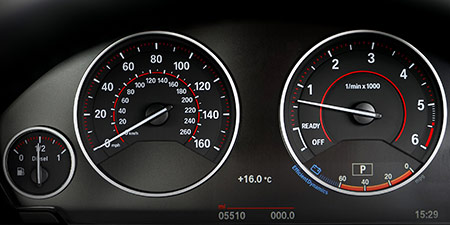# Miles per hour (mph) to Miles per second (mi/s)

Units of speed ﹣ Converter Miles per hour to Miles per second

Here you can convert the Speed unit Miles per hour into the unit Miles per second and vice versa you can convert Miles per second into Miles per hour. By clicking the "Swap units" icon, you will always obtain the desired conversion in the calculation result, i.e., mph to mi/s or mi/s to mph. With the following calculator you can also calculate any other Speed unit.

## Info about "Miles per hour"

The unit of length known as a "mile" and it should not be confused with the nautical mile and very commonly used in the Anglo-American countries. Therefore, the metric system is defined in miles (instead of kilometers) per time unit. For example, if a vehicle travels at a speed of 100 miles per hour (100 mph), it will move around 160 km per hour in the metric system.

The units are defined as follows: An object moving at 1 "mph" per hour will cover a distance of one mile. Therefore, 1 mile is equivalent to 1.609344 kilometers.

## Info about "Miles per second"

The length unit "mile" is commonly used in the Anglo-American countries, where speeds are represented in miles (instead of kilometers) per time unit and it should not be confused sea mile.

The units are defined as follows: An object moving at 1 "mph" per hour will cover a distance of one mile. Therefore, 1 mile is equivalent to 1.609344 kilometers.

## Basis for conversion Miles per hour (mph) to Miles per second (mi/s) and vice versa

The abbreviation for the "Speed unit Miles per hour" is mph. The abbreviation for the "Speed unit Miles per second" is mi/s.

## Formula for the conversion of Miles per hour (mph) to Miles per second (mi/s) and vice versa

The calculation from Miles per hour to Miles per second shall be made using the following conversion formula:

Conversion formula Miles per hour to Miles per second

Determine the number of Miles per second from Miles per hour

Miles per hour × 0.00027777777766602

## Formula for the conversion of Miles per second (mi/s) to Miles per hour (mph)

The calculation from Miles per second to Miles per hour shall be made using the following conversion formula:

Conversion formula Miles per second to Miles per hour

Determine the number of Miles per hour from Miles per second

Miles per second × 3600

## Overview table : How many Miles per hour are in a Miles per second ?

Miles per hour mph ⇒ Miles per second mi/s
0.04 mph  are  0.00001 mi/s
0.05 mph  are  0.00001 mi/s
0.06 mph  are  0.00001 mi/s
0.07 mph  are  0.00001 mi/s
0.08 mph  are  0.00002 mi/s
0.09 mph  are  0.00002 mi/s
0.10 mph  are  0.00002 mi/s
0.20 mph  are  0.00005 mi/s
0.30 mph  are  0.00008 mi/s
0.40 mph  are  0.00011 mi/s
0.50 mph  are  0.00013 mi/s
0.60 mph  are  0.00016 mi/s
0.70 mph  are  0.00019 mi/s
0.80 mph  are  0.00022 mi/s
0.90 mph  are  0.00025 mi/s
1 mph  corresponds to  0.00027 mi/s
2 mph  are  0.00055 mi/s
3 mph  are  0.00083 mi/s
4 mph  are  0.00111 mi/s
5 mph  are  0.00138 mi/s
6 mph  are  0.00166 mi/s
7 mph  are  0.00194 mi/s
8 mph  are  0.00222 mi/s
9 mph  are  0.00250 mi/s
10 mph  are  0.00277 mi/s
20 mph  are  0.00555 mi/s
30 mph  are  0.00833 mi/s
40 mph  are  0.01111 mi/s
50 mph  are  0.01388 mi/s
60 mph  are  0.01666 mi/s
70 mph  are  0.01944 mi/s
80 mph  are  0.02222 mi/s
90 mph  are  0.02500 mi/s
100 mph  are  0.02777 mi/s
200 mph  are  0.05555 mi/s
300 mph  are  0.08333 mi/s
400 mph  are  0.11111 mi/s
500 mph  are  0.13888 mi/s
600 mph  are  0.16666 mi/s
700 mph  are  0.19444 mi/s
800 mph  are  0.22222 mi/s
900 mph  are  0.25000 mi/s
1 000 mph  are  0.27777 mi/s

## Overview table : How many Miles per second are in a Miles per hour ?

Miles per second mi/s ⇒ Miles per hour mph
0.01 mi/s  are  36 mph
0.02 mi/s  are  72 mph
0.03 mi/s  are  108 mph
0.04 mi/s  are  144 mph
0.05 mi/s  are  180 mph
0.06 mi/s  are  216 mph
0.07 mi/s  are  252 mph
0.08 mi/s  are  288 mph
0.09 mi/s  are  324 mph
0.10 mi/s  are  360 mph
0.20 mi/s  are  720 mph
0.30 mi/s  are  1 080 mph
0.40 mi/s  are  1 440 mph
0.50 mi/s  are  1 800 mph
0.60 mi/s  are  2 160 mph
0.70 mi/s  are  2 520 mph
0.80 mi/s  are  2 880 mph
0.90 mi/s  are  3 240 mph
1 mi/s  corresponds to  3 600 mph
2 mi/s  are  7 200 mph
3 mi/s  are  10 800 mph
4 mi/s  are  14 400 mph
5 mi/s  are  18 000 mph
6 mi/s  are  21 600 mph
7 mi/s  are  25 200 mph
8 mi/s  are  28 800 mph
9 mi/s  are  32 400 mph
10 mi/s  are  36 000 mph
20 mi/s  are  72 000 mph
30 mi/s  are  108 000 mph
40 mi/s  are  144 000 mph
50 mi/s  are  180 000 mph
60 mi/s  are  216 000 mph
70 mi/s  are  252 000 mph
80 mi/s  are  288 000 mph
90 mi/s  are  324 000 mph
100 mi/s  are  360 000 mph
200 mi/s  are  720 000 mph
300 mi/s  are  1 080 000 mph
400 mi/s  are  1 440 000 mph
500 mi/s  are  1 800 000 mph
600 mi/s  are  2 160 000 mph
700 mi/s  are  2 520 000 mph
800 mi/s  are  2 880 000 mph
900 mi/s  are  3 240 000 mph
1 000 mi/s  are  3 600 000 mph